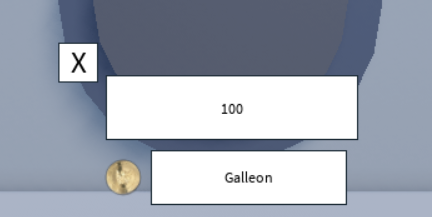# In-game Transaction

Hello, I’m trying to make my users be able to deposit their in-game currency into their in-game bank vault. In my game I have three currencies: Galleons, Sickles and Knuts so I made a GUI that lets them choose the currency they want to deposit.

When they press enter after writing the amount of that currency they want to deposit, it should add to their vault that amount and decrease the current user’s amount, for that I use this code:

``````local TS = game:GetService("TweenService")

local RS = game:GetService("ReplicatedStorage")
local GringottsDir = RS.Gringotts
local RemoteEvents = GringottsDir.RemoteEvents

local DepositTransactionRE = RemoteEvents.DepositTransaction

DepositTransactionRE.OnServerEvent:Connect(function(Player, value, currencyType)
-- TODO: Transaction
return
end
end)
``````

This is a server script.

I also have a localscript:

``````script.Parent.Second.DepositButton.MouseButton1Click:Connect(function()
local Children = script.Parent.Second:GetChildren()

for i = 1, #Children do
if Children[i].Name ~= "DepositTextBox" or "DepositCloseButton" then
Children[i].Visible = false
end
end

script.Parent.Second.DepositTextBox.Visible = true
script.Parent.Second.DepositCloseButton.Visible = true

script.Parent.Second.DepositTextBox.FocusLost:Connect(function(key)
local text = script.Parent.Second.DepositTextBox.Text
print("HELLO TEXT ISNT NIL")
if key then
print("HELLO KEY ISNT NIL")
if tonumber(text) then
print("tonumber")
local value = tonumber(text)
local currencyType

print("before for :(")
for i = 1, #currencies do
print("?")
if script.Parent.Second.DepositTextBox.DepositTextBox.Text == "" then
print("a")
end
if script.Parent.Second.DepositTextBox.DepositTextBox.Text == currencies[i].Name then
print("transaction")
-- TODO: Transaction
currencyType = script.Parent.Second.DepositTextBox.DepositTextBox.Text
DepositTransactionRE:FireServer(value, currencyType)
print("after transaction")
end
end
end
end
end)
end)
``````

But none of the prints work, and I get no errors from the console nor the transaction gets completed.

I have already tried remaking the GUI, changing how the script works (before it was in StarterPlayer, now it is in a folder in StarterGui) and using prints to try to check where was the issue. Nothing worked.

(Btw, before adding the currency types selection, the transaction worked correctly, so it isn’t a problem that had the original code).

This is how the GUI looks:Explorer View:try this. I optimized/rewrote some of your code.

``````local DepositTextBox = script.Parent.Second.DepositTextBox
local DepositButton = script.Parent.Second.DepositButton
local DepositCloseButton = script.Parent.Second.DepositButton
-- Makes it so the input only accepts numbers (thought this may be helpful to include)
DepositTextBox:GetPropertyChangedSignal("Text"):Connect(function()
DepositTextBox.Text = DepositTextBox.Text:gsub('%D+', '');
end

DepositTextBox.FocusLost:Connect(function(enterPressed)
local text = DepositTextBox.Text

if (text == "" or tonumber(text) <= 0)  then warn("Number was 0 or nothing was entered")  return end)

print("Text is not empty or equal to 0")
if enterPressed  then
print("Enter was pressed")
if tonumber(text) then
print("tonumber")
local value = tonumber(text)
local currencyType

print("before for :(")
for i = 1, #currencies do
print("?")
if script.Parent.Second.DepositTextBox.DepositTextBox.Text == "" then
print("a")
end
if script.Parent.Second.DepositTextBox.DepositTextBox.Text == currencies[i].Name then
print("transaction")
-- TODO: Transaction
currencyType = script.Parent.Second.DepositTextBox.DepositTextBox.Text
DepositTransactionRE:FireServer(value, currencyType)
print("after transaction")
end
end
end
end
end)

DepositButton.MouseButton1Click:Connect(function()
local Children = script.Parent.Second:GetChildren()

for i = 1, #Children do
if Children[i].Name ~= "DepositTextBox" or "DepositCloseButton" then
Children[i].Visible = false
end
end

DepositTextBox.Visible = true
DepositCloseButton.Visible = true
end)

DepositCloseButton.MouseButton1Click:Connect(function()
local Children = script.Parent.Second:GetChildren()

for i = 1, #Children do
if Children[i].Name ~= "DepositTextBox" or "DepositCloseButton" then
Children[i].Visible = true
end
end

DepositTextBox.Visible = false
DepositCloseButton.Visible = false
end)
``````

I found the issue, but I’ll still use your code, tysm, I’ll mark your comment as the solution.